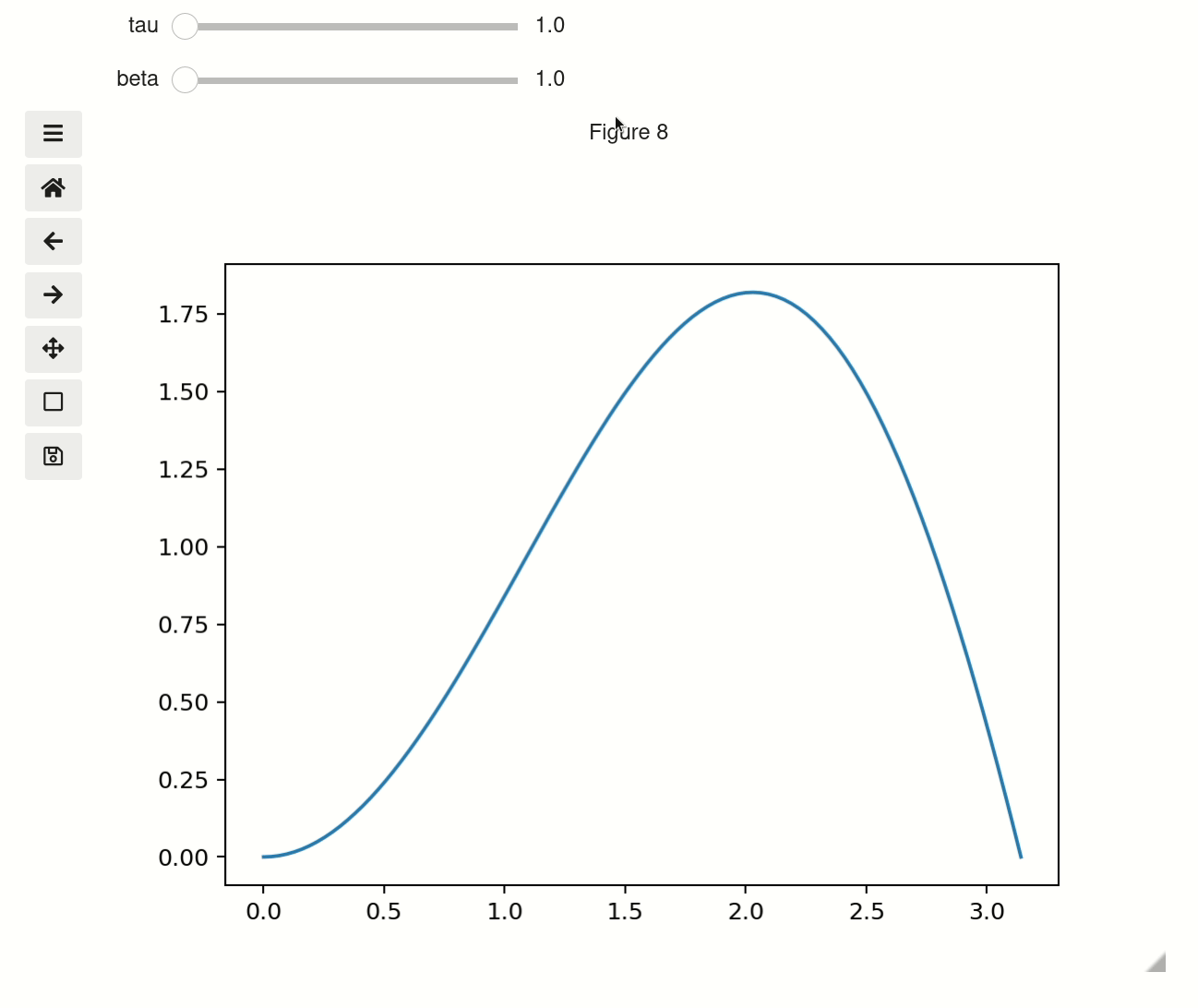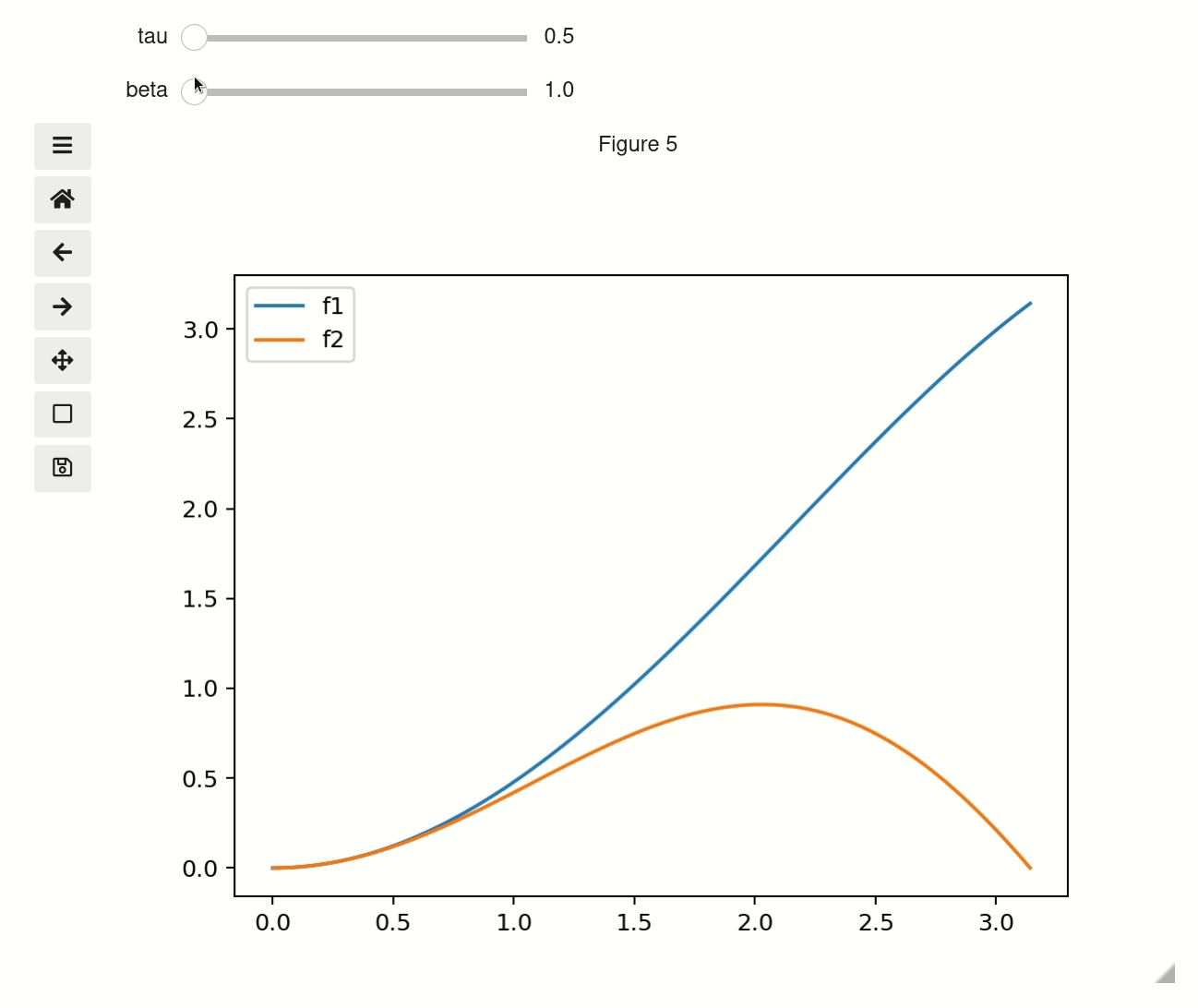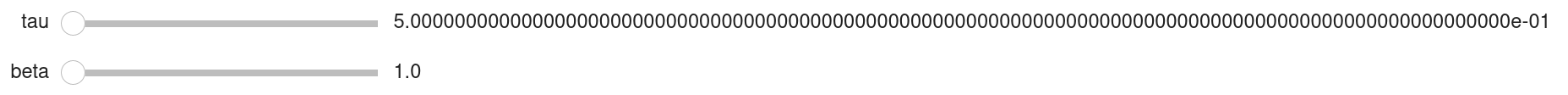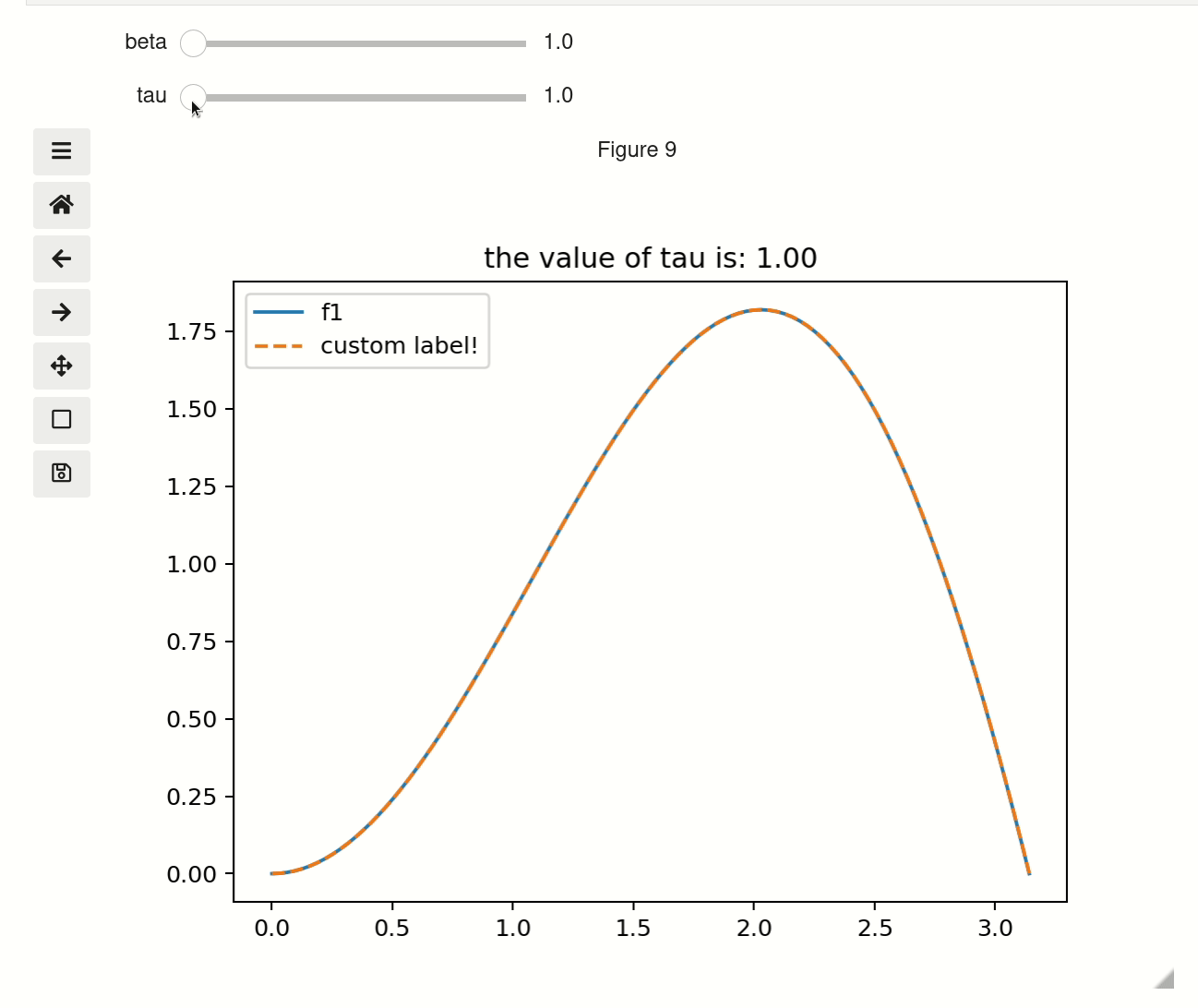# Line plots¶

Note

Unfortunately the interactive plots won’t work on a website as there is no Python kernel running. So for this site all the interactive outputs have been replaced by gifs of what you will see.

On this example page all of the outputs will use ipywidgets widgets for controls. If you are not working in a Jupyter Notebook the examples here will still work with the built-in Matplolitb widgets. For examples that that explicitly use Matplotlib widgets instead of ipywidgets see the Using Matplotlib Widgets page.

```# only run these lines if you are using a Jupyter Notebook or JupyterLab
%matplotlib ipympl
import ipywidgets as widgets

# rest of imports
import matplotlib.pyplot as plt
import numpy as np
from mpl_interactions import interactive_plot
```

## Simple example¶

To use the interactive plot function, write a function that will return a NumPy array or a list of numbers. You can provide the parameters you want to vary with sliders as keyword arguments to the `interactive_plot()` function.

```x = np.linspace(0, np.pi,100)
tau = np.linspace(1,10, 100)
beta = np.linspace(1,10)
def f(x, tau, beta):
return np.sin(x*tau)*x**beta
```

Then to display the plot:

```fig, ax, sliders = interactive_plot(f, x=x, tau = tau, beta = beta)
```## Other ways to set parameter values¶

You can set parameters using any of the following:

• NumPy array/list - Creates a slider with the values in the array

• tuple - Acts as an argument to linspace (can have either 2 or 3 items)

• set - Creates a categorical selector (order will not preserved)

• set(tuple()) - Categorical selector with order maintained

• scalar - Fixed value

• ipywidgets.Widget - any subclass of `ipywidgets.Widget` that has a `value` attribute can be used

• matplotlib.widgets.Slider or RadioButton - Note this cannot be used at the same time as an `ipywidgets.Widget`

Here is an example using all of the possibilities with a dummy function.

Note

The slider labels will not update here as that update requires a Python kernel.

Also, `display=False` prevents the widgets from being automatically displayed, making it easier to render them on this webpage. In general you should not need to use it.

```def foo(x, **kwargs):
return x

a = np.linspace(0,10)
b = (0, 10, 15)
c = {'this', 'set will be', 'unordered'}
d = {('this', 'set will be', 'ordered')}
e = 0 # this will not get a slider
f = widgets.Checkbox(value=True, description='A checkbox!!')
display(interactive_plot(foo, x=x, a=a, b=b, c=c, d=d, e=e, f_=f, display=False)[-1])
```

## Multiple functions¶

To plot multiple functions simply pass a list of functions as the first argument `interactive_plot([f1, f2],...)`. When you add a legend to the resulting plot, the function names will be used as the labels unless overriden using the plot_kwargs argument.

```def f1(x, tau, beta):
return np.sin(x*tau)*x*beta
def f2(x, tau, beta):
return np.sin(x*beta)*x*tau
fig, ax, sliders = interactive_plot([f1, f2], x=x, tau = tau, beta = beta, display=False)
_ = plt.legend()
```## Styling¶

Calling `interactive_plot` will create and display a new figure. Then you can either use the standard `pyplot` command to continue modifying the plot, or you can use the references to the `figure` and `axis` that are returned by `interactive_plot`. Though be careful, anything you add will not be affected by the sliders.

### Slider precision¶

You can change the precision of individual slider displays by passing `slider_format_string` as a dictionary. The example below gives the tau slider 99 decimal points of precision and uses scientific notation to display it. The beta slider uses the default 1 decimal point of precision.

```interactive_plot(f, x=x, tau=tau, beta=beta, slider_format_string = {"tau": '{:.99e}'})
```### Axis limits¶

You can control how the `xlim/ylim` behaves using the `xlim/ylim` arguments. The options are:

1. `'stretch'` - The default; allows the x/y axes to expand but never shrink

2. `'auto'` - Autoscales the limits for every plot update

3. `'fixed'` - Never automatically update the limits

4. [`float`, `float`] - This value will be passed through to `plt.xlim` or `plt.ylim`

### Reference parameter values in the Title¶

You can make the Title automatically update with information about the values by using `title` argument. Use the name of one of the parameters as a format specifier in the string. For example use the following title string to put the value of tau in the title and round it to two decimalsg: `{'tau:.2f}'`

### Matplolitb keyword arguments¶

You can pass keyword arguments (kwargs) through to the `plt.plot` calls using the `plot_kwargs` argument to `interactive_plot`. For example, to add a label and some styling to one of the functions try the following:

```interactive_plot([f1, f2], x=x, beta=beta, tau=tau,
plot_kwargs=[{}, {'label':'custom label!', 'linestyle':'--'}],
title='the value of tau is: {tau:.2f}'))
```